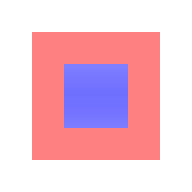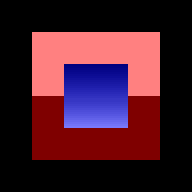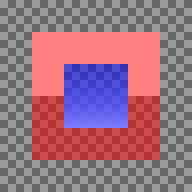First publication
2019-04-18
Last update
2019-04-18

I am currently working on an SWF renderer. The renderer supports well-formed shapes but the spec does not describe how to handle self-intersections, non-closed paths, invalid styles, etc. To generate more test cases, my idea is to use Adobe's Flash player as a reference implementation: pass the shape definitions to it and take screenshots.

The main issue with this method is that SWF shapes can have transparency (an alpha channel) while screenshots are opaque. This post explains my method to retrieve the alpha layer using multiple screenshots.

Below is an example shape. When drawn on top of a white background, it looks like a simple light-blue square with a pink border. When drawn on top of a black background, we see that the shape is in fact more complex. Using these two screenshots, we can retrieve the alpha value and real colors.## Alpha compositing

We first need to understand how the background an image are merged together in the screenshots before we try to reverse this process. This operation is called alpha compositing.

In computer graphics, alpha compositing is the process of combining an image with a background to create the appearance of partial or full transparency

Alpha compositing - Wikipedia

There are many ways to describe an opaque color but most commonly we use an RGB tuple. The color is described by the intensity of its red, green and blue components (or channels). The values are often integers between 0 and 255 but in this article I'll use ratios between 0 and 1 because it simplifies the expressions (this is also how the colors are represented in the GPU).

The alpha channel is an extra component used to represent the opacity of a color. 0 means that the color is fully transparent, 1 that it is fully opaque.

Here are some examples:

\begin{align} C_\text{white} &= (1, 1, 1, 1) \\ C_\text{black} &= (0, 0, 0, 1) \\ C_\text{semiTransparentRed} &= (1, 0, 0, 0.5) \\ C_\text{pink} &= (1, 0.5, 0.5, 1) \end{align}

When blending a partially-transparent color $C_\text{front}$ on top of an opaque background $C_\text{back}$, the result is an opaque weighted average $C_\text{result}$. $C_\text{front}$ has a weight $\alpha$ and $C_\text{back}$ has the remaining weight $(1 - \alpha)$.

\begin{align} r_\text{result} &= \alpha r_\text{front} + (1 - \alpha) r_\text{back} \\ g_\text{result} &= \alpha g_\text{front} + (1 - \alpha) g_\text{back} \\ b_\text{result} &= \alpha b_\text{front} + (1 - \alpha) b_\text{back} \\ \end{align}

Here is an example with $C_\text{semiTransparentRed}$ on top of $C_\text{white}$:

\begin{align} r_\text{result} &= 0.5 \times 1 + (1 - 0.5) 1 = 1\\ g_\text{result} &= 0.5 \times 0 + (1 - 0.5) 1 = 0.5\\ b_\text{result} &= 0.5 \times 0 + (1 - 0.5) 1 = 0.5\\ \end{align}

We get an opaque $C_\text{pink}$. This is why the example has a uniform pink border when rendered on top of a white background.## Extracting alpha

We can control the background color and read the result, the unknown values are the color and alpha channels of the front color.

Each background gives one equation per color channel. One background is not enough: 4 unknowns for only 3 equations. We need to test at least two different backgrounds to get enough information.

Let define the unknown front color as $C = (r, g, b, \alpha)$ and the background colors $C_{bi} = (r_{bi}, g_{bi}, b_{bi}, 1)$ and corresponding results $C_{ri} = (r_{ri}, g_{ri}, b_{ri}, 1)$.

With two background/result pairs, we have the following 6 equations:

\begin{align} r_\text{r0} &= \alpha r + (1 - \alpha) r_\text{b0} \\ r_\text{r1} &= \alpha r + (1 - \alpha) r_\text{b1} \\ g_\text{r0} &= \alpha g + (1 - \alpha) g_\text{b0} \\ g_\text{r1} &= \alpha g + (1 - \alpha) g_\text{b1} \\ b_\text{r0} &= \alpha b + (1 - \alpha) b_\text{b0} \\ b_\text{r1} &= \alpha b + (1 - \alpha) b_\text{b1} \end{align}

Each channel allows to retrieve the alpha value. By taking the difference between each pair we get rid of of $\alpha r$ / $\alpha g$ / $\alpha b$ factor and can isolate $\alpha$:

\begin{align} \alpha &= 1 - { {r_\text{r1} - r_\text{r0}} \over {r_\text{b1} - r_\text{b0}} } \\ \alpha &= 1 - { {g_\text{r1} - g_\text{r0}} \over {g_\text{b1} - g_\text{b0}} } \\ \alpha &= 1 - { {b_\text{r1} - b_\text{r0}} \over {b_\text{b1} - b_\text{b0}} } \end{align}

We have the choice between 3 equations: which one should we use? If we ignore the precision of the values and computations, it does not matter. Either they all have the same value, or the background/result pairs were invalid (didn't correspond to alpha compositing). Unfortunately, computers don't have infinite precision: we'll discuss precision in the next section.

Once we know $\alpha$, any equation can give its corresponding color value:

\begin{align} r &= { {r_\text{r0} - (1 - \alpha) r_\text{b0}} \over { \alpha } } \\ r &= { {r_\text{r1} - (1 - \alpha) r_\text{b1}} \over { \alpha } } \\ g &= { {g_\text{r0} - (1 - \alpha) g_\text{b0}} \over { \alpha } } \\ g &= { {g_\text{r1} - (1 - \alpha) g_\text{b1}} \over { \alpha } } \\ b &= { {b_\text{r0} - (1 - \alpha) b_\text{b0}} \over { \alpha } } \\ b &= { {b_\text{r1} - (1 - \alpha) b_\text{b1}} \over { \alpha } } \end{align}

Again, there are multiple expressions for the same variable and they should all evaluate to the same value. What if $\alpha = 0$? Then the color is fully transparent so we don't care about the color components: any value can be used.

Here is an example reversing a pixel from the bottom border.On a white background $C_\text{b0} = (1, 1, 1, 1)$, the result is pink $C_\text{r0} = (1, 0.5, 0.5, 1)$. On a black background $C_\text{b1} = (0, 0, 0, 1)$, the result is dark red $C_\text{r1} = (0.5, 0, 0, 1)$.

We get the following values for $\alpha$:

\begin{align} \alpha &= 1 - { {0.5 - 1} \over {0 - 1} } = 0.5 \\ \alpha &= 1 - { {0 - 0.5} \over {0 - 1} } = 0.5 \\ \alpha &= 1 - { {0 - 0.5} \over {0 - 1} } = 0.5 \end{align}

They all match: we can be confident that the alpha value is $0.5$.

We can then compute the color components:

\begin{align} r &= { {1 - (1 - 0.5) 1} \over { 0.5 } } = 1\\ r &= { {0.5 - (1 - 0.5) 0} \over { 0.5 } } = 1\\ g &= { {0.5 - (1 - 0.5) 1} \over { 0.5 } } = 0\\ g &= { {0 - (1 - 0.5) 0} \over { 0.5 } } = 0\\ b &= { {0.5 - (1 - 0.5) 1} \over { 0.5 } } = 0\\ b &= { {0 - (1 - 0.5) 0} \over { 0.5 } } = 0 \end{align}

The color components agree. We now know the true color of the border: $(1, 0, 0, 0.5)$ (semi-transparent red).

## Precision

The previous section assumed mathematical values: colors with infinite precision. There were many redundant equations but they all led to the same value anyway so it did not matter which one we choose.

Now, computers have a finite precision so we may get slightly different results based on the expression we choose. How to chose the best one?

To help us decide, let's check how errors propagate and then choose the most precise result. The background color is an input we control: the value is discrete but there is no error. During the blending, we'll assume that the colors are handled as floating points: they have a pretty large precision so we can assume there is no precision loss there. This result is then written to an 8-bit channel: this is were the largest error appear. If we assume that result is rounded to the nearest value, it means that we have an error $\epsilon = {0.5 \over 2^8}$ and a component $x$ in the result color corresponds in fact to the semi-open range $[{x_{min} = x - \epsilon}; {x_{max} = x+\epsilon}[$.

For the channel $x$, we had the following equation for the alpha value:

\begin{align} \alpha &= 1 - { {x_\text{r1} - x_\text{r0}} \over {x_\text{b1} - x_\text{b0}} } \end{align}

Using the min and max values for $x_\text{r0}$ and $x_\text{r1}$, we can get the range for $\alpha$. Note: this model assumes that $x_\text{r0}$ and $x_\text{r1}$ are independent so the range we get may overestimate the error on $\alpha$.

\begin{align} \alpha_{min} &= 1 - { {x_\text{r1 max} - x_\text{r0 min}} \over {x_\text{b1} - x_\text{b0}} } \\ &= 1 - { {x_\text{r1} - x_\text{r0} + 2\epsilon} \over {x_\text{b1} - x_\text{b0}} } \\ &= \alpha - { {2\epsilon} \over {x_\text{b1} - x_\text{b0}} } \\ \alpha_{max} &= 1 - { {x_\text{r1 min} - x_\text{r0 max}} \over {x_\text{b1} - x_\text{b0}} } \\ &= 1 - { {x_\text{r1} - x_\text{r0} - 2\epsilon} \over {x_\text{b1} - x_\text{b0}} } \\ &= \alpha + { {2\epsilon} \over {x_\text{b1} - x_\text{b0}} } \end{align}

We get that the error on $\alpha$ is ${2\epsilon} \over {x_\text{b1} - x_\text{b0}}$. It confirms the intuition that we get smaller errors when input backgrounds are more different. When using black and white backgrounds, the error corresponds to 1 bit.

Using 8-bit integers, if we use black and white backgrounds and get $\alpha = 234.7$, it means that the real value is either $\alpha = 234$ or $\alpha = 235$. The equations from the 3 channels should intersect on at least one integer, the real value. If they intersect on two values, the closest to the average should be preferred. If the ranges do not intersect over an integer, the values are invalid.

Using black and white background and by fixing $\alpha$ at this point, we have either the true $\alpha$ value or the result was invalid. It means the alpha value we use to retrieve the color components is not tainted by error.

For the color $x$, we have the following equations:

\begin{align} x_0 &= { {x_\text{r0} - (1 - \alpha) x_\text{b0}} \over { \alpha } } \\ x_1 &= { {x_\text{r1} - (1 - \alpha) x_\text{b1}} \over { \alpha } } \\ \end{align}

We can compute the ranges using the error on $x_\text{r0}$ and $x_\text{r1}$:

\begin{align} x_\text{0 min} &= { {x_\text{r0 min} - (1 - \alpha) x_\text{b0}} \over { \alpha } } \\ &= { {x_\text{r0} - \epsilon - (1 - \alpha) x_\text{b0}} \over { \alpha } } \\ &= x_0 - { \epsilon \over \alpha } \\ x_\text{0 max} &= { {x_\text{r0 max} - (1 - \alpha) x_\text{b0}} \over { \alpha } } \\ &= { {x_\text{r0} + \epsilon - (1 - \alpha) x_\text{b0}} \over { \alpha } } \\ &= x_0 + { \epsilon \over \alpha } \\ x_\text{1 min} &= x_1 - { \epsilon \over \alpha } \\ x_\text{1 max} &= x_1 + { \epsilon \over \alpha } \\ \end{align}

For the color components, the error is $\epsilon \over \alpha$.

Again, we can intersect all the ranges and restrict the possible solutions to integers. If $alpha >= 0.5$, the error is smaller than 1 bit and there should be 1 or 2 integer solutions. For small values, when the color is almost fully transparent, the error can get quite large (up to $0.5$).

## Conclusion

The best way to extract alpha from screenshots is to use black and white backgrounds.

If the result color matches the background color, the real color is fully transparent.

Otherwise, we can compute the alpha value using the red, green or blue channels:

\begin{align} \alpha &= 1 - { {x_\text{r1} - x_\text{r0}} \over {x_\text{b1} - x_\text{b0}} } \end{align}

By averaging the values and choosing the closest integer, we get the real alpha value.

For each color channel, we get one value from the black background and one value from the white background:

\begin{align} x_\text{black} &= { {x_\text{r black} } \over { \alpha } } \\ x_\text{white} &= 1 - { {1 - x_\text{r white}} \over { \alpha } } \\ \end{align}

These values have an error of $\epsilon \over \alpha$ where $\epsilon = {0.5 \over 2^8}$. The color value is the integer closest to the average.